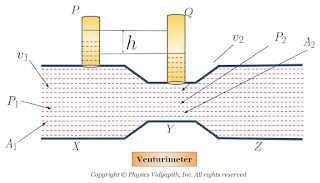### Principle, Construction and Working of Venturimeter

Principle of Venturimeter:

It is a device that is used for measuring the rate of flow of liquid through pipes. Its principle and working are based on Bernoulli's theorem and equation.

Construction:

It consists of two identical coaxial tubes $X$ and $Z$ connected by a narrow co-axial tube $Y$. Two vertical tubes $P$ and $Q$ are mounted on tubes $X$ and $Y$ to measure the pressure of the liquid that flows through pipes.. As shown in the figure below.Working and Theory:

Connect this venturimeter horizontally to the pipe through which the liquid is flowing and note down the difference of liquid columns in tubes $P$ and $E$. Let the difference is $h$.

Let us consider that an incompressible and non-viscous liquid flows in streamlined motion through a tube $X$,$Y$, and $Z$ of the non-uniform cross-section.

Now Consider:

The Area of cross-section of tube $X$ = $A_{1}$
The Area of cross-section of tube $Y$ = $A_{2}$

The velocity per second (i.e. equal to distance) of fluid at the cross-section of tube $X$ = $v_{1}$
The velocity per second (i.e. equal to distance) of fluid at the cross-section of tube $Y$ = $v_{2}$

The Pressure of fluid at cross-section of tube $X$ = $P_{1}$
The Pressure of fluid at cross-section of tube $Y$ = $P_{2}$

Now the change in pressure on tube $P$ and $Q$:

$P_{1}-P_{2}=\rho g h \qquad(1)$

Where $\rho$ is the density of liquid.

According to the principle of continuity:

$A_{1} v_{1} = A_{2} v_{2} = \frac{m}{\rho}= V \qquad(2)$

Where $V$ is the volume of the liquid flowing per second through the pipe. Then from equation $(2)$

$\left.\begin{matrix} v_{1}=\frac{V}{A_{1}} \\ v_{2}=\frac{V}{A_{2}} \end{matrix}\right\} \qquad(3)$

Now apply Bernoulli's Theorem for horizontal flow (i.e $h_{1}=h_{2}$) in verturimeter.

$P_{1} + \frac{1}{2} \rho v_{1}^{2} = P_{2} + \frac{1}{2}\rho v_{2}^{2}$

$P_{1} - P_{2} = \frac{1}{2}\rho v_{2}^{2} - \frac{1}{2} \rho v_{1}^{2}$

$P_{1} - P_{2} = \frac{1}{2}\rho \left( v_{2}^{2} - v_{1}^{2} \right) \qquad(4)$

Now from equation $(1)$ and equation $(4)$, we get

$\rho g h = \frac{1}{2}\rho \left( v_{2}^{2} - v_{1}^{2} \right)$

$g h = \frac{1}{2} \left( v_{2}^{2} - v_{1}^{2} \right)$

Now substitute the value of $v_{1}$ and $v_{2}$ from equation $(3)$ in above equation

$g h = \frac{1}{2} \left( \frac{V^{2}}{A^{2}_{2}} - \frac{V^{2}}{A^{2}_{1}} \right)$

$g h = \frac{V^{2}}{2} \left( \frac{1}{A^{2}_{2}} - \frac{1}{A^{2}_{1}} \right)$

$g h = \frac{V^{2}}{2} \left( \frac{ A^{2}_{1} - A^{2}_{2} }{A^{2}_{1} A^{2}_{2}} \right)$

$V^{2} = \frac{2 g h A^{2}_{1} A^{2}_{2}}{A^{2}_{1} - A^{2}_{2}}$

$V = A_{1} A_{2} \sqrt{\frac{2 g h }{A^{2}_{1} - A^{2}_{2}}}$

Hence flow rate of liquid can be calculated by measuring $h$, since $A_{1}$ and $A_{2}$ are known for the given venturimeter.